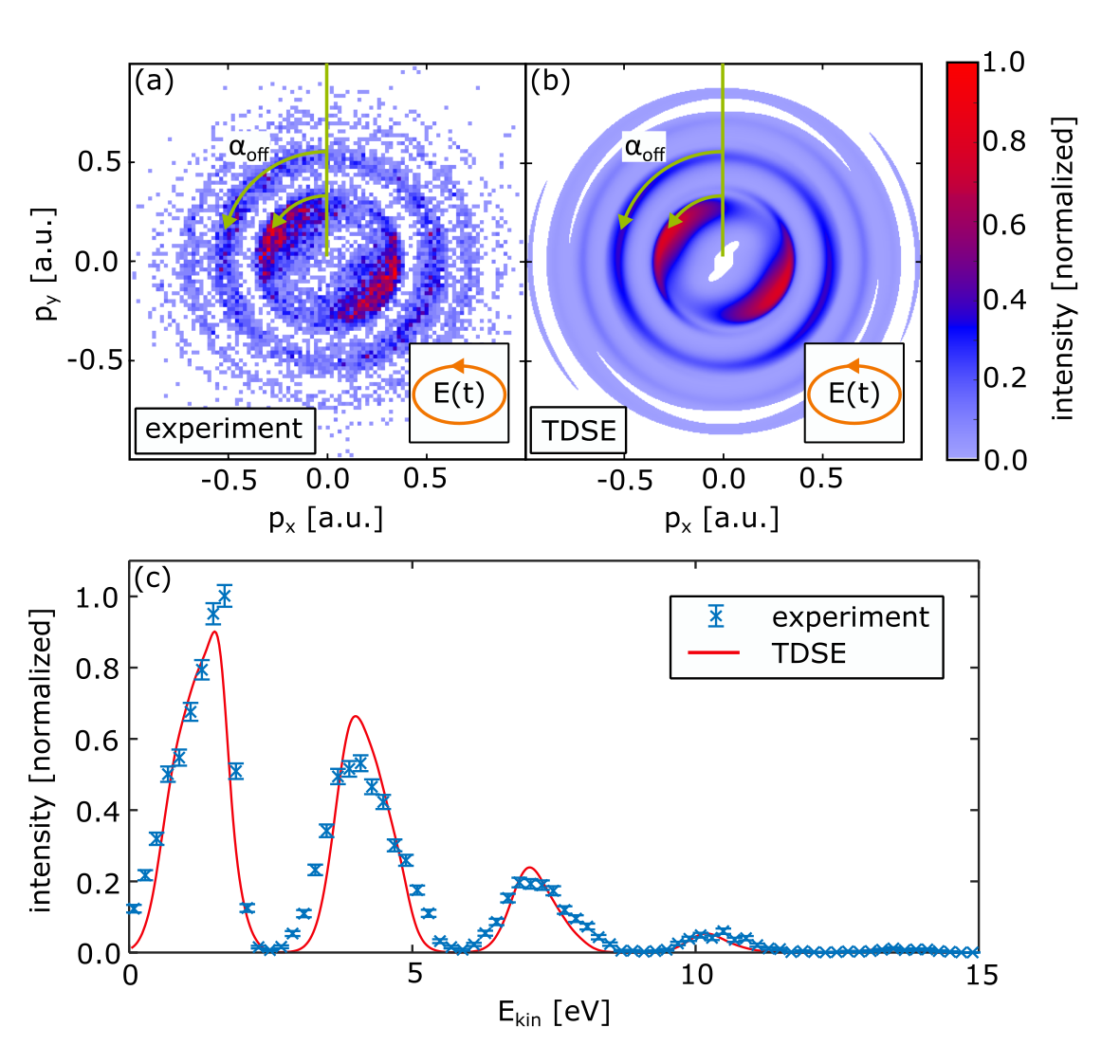﻿ ﻿Atomic Physics - Non-adiabatic Strong Field Ionization of Atomic Hydrogen
 Atomic hydrogen is the simplest atomic system and thus it is often used to benchmark theoretical models, making it the drosophila of theories of light-matter interaction. Its key advantage is the absence of multielectron effects and the well-defined electrostatic potential of the proton, eliminating the need for approximations. Atomic hydrogen is very frequently used in theoretical studies and textbook examples. The experimental challenges connected with producing hydrogen atoms in an ultrahigh vacuum environment and separating events from those resulting from nondissociated hydrogen molecules make it difficult to study it using strong laser fields.  Here, we present experimental data on the nonadiabatic strong field ionization of atomic hydrogen using elliptically polarized femtosecond laser pulses at a central wavelength of 390 nm. A COLTRIMS reaction microscope was used to measure the three-dimensional momenta of electron and ion in coincidence. FIG. 1(a) shows the electron momentum distribution projected onto the laser’s polarization plane (pxpy plane). FIG. 1(b) shows the corresponding result that is obtained from a numerical solution of the time-dependent Schrödinger equation (TDSE). Experiment and TDSE result are in excellent agreement. This is underlined by FIG. 1(c), which shows the corresponding electron energy distributions.  As can be seen in FIG. 2(a) and 2(b), the most probable emission angle (also known as “attoclock offset angle” or “streaking angle”) αoff is found to increase with energy. This trend is opposite to standard predictions based on Coulomb interaction with the ion as described by the classical two-step (CTS) model shown in FIG. 2(c). The increase of deflection angle can be explained by the nonadiabatic, classical two-step (NACTS) model that includes nonadiabatic corrections of the initial momentum distribution at the tunnel exit and nonadiabatic corrections of the tunnel exit position itself. The prediction of the NACTS model is shown in FIG. 2(d) and reproduces the same trend as observed in the experiment and the TDSE simulation.  We expect that the NACTS model will help to interpret future attoclock experiments and will thereby lead to a better understanding of the role of electron positions and momenta in the ionization process.FIG. 1. (a) Measured electron momentum distribution projected onto the laser’s polarization plane for the ionization of atomic hydrogen by femtosecond laser pulses at a central wavelength of 390 nm, an ellipticity of ε = 0.85, and a peak intensity of 1.4 × 1014 W/cm2. The light’s helicity and the orientation of the polarization ellipse are indicated by the inset in the lower right corner. (b) Focal-averaged numerical solution of the TDSE for the parameters that were used in (a). αoff indicates the “angular offset” with respect to the minor axis of the ellipse of the laser electric field and the negative vector potential in (a) and (b). The intensity in (a) [(b)] has been normalized to a maximum of 1 [0.8]. (c) shows a comparison of the electron energy distribution for the data shown in (a) and (b). The error bars show statistical errors only.FIG. 2. (a) [(b)] shows the electron momentum distribution from Fig. 1(a) [Fig. 1(b)] in cylindrical coordinates after rowwise normalization. αoff indicates the angular offset, the white contours indicate the ATI peak positions. (c) The rowwise normalized electron momentum distribution that is obtained from the CTS model with initial momenta distributed according to ADK theory, taking Coulomb interaction after tunneling into account and using the tunnel exit position from the TIPIS model in analogy to (a) and (b). (d) The result from our NACTS model. Within the NACTS model, the ionization probability and the initial conditions are taken from SFA. The black line guides the eye and is the same in all panels. The data in (a)–(d) have been symmetrized making use of the twofold symmetry. Every row has been normalized independently. The intensity in (a) [(b)–(d)] has been normalized to a maximum of 1 [0.8].  Publication: Nonadiabatic Strong Field Ionization of Atomic Hydrogen D. Trabert, N. Anders, S. Brennecke, M. S. Schöffler, T. Jahnke, L. Ph. H. Schmidt, M. Kunitski, M. Lein, R. Dörner, and S. Eckart DOI: 10.1103/PhysRevLett.127.273201 Phys. Rev. Lett. (2021)  See online: Phys. Rev. Lett. arXiv